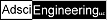#### Hypergeometric Probability Distribution Calculator (ALPHA)Enter Size of Space Enter number of desirables Enter number of undesirables Sample Size Start Stop

• The "Space" is the sample space, i.e. the number of marbles in bag in the bag of marbles example
• The "Desirables" are the number of desirable objects within the space, so, if you have a bag of marbles, and 30 of them are red and 60 of them are blue, and you want a red one, 30 would be your "desirables" number
• The "Undesirables" are the number of undesirable objects within the space, i.e. the blue marbles
• It is only necessary to specify either the desirables or the undesirables, not both, since they are assumed to be complimentary.
• The "sample" is the number of objects you are extracting from the space without replacement to consider statistically. "Take x marbles from the bag."
• The "Start" and "Stop" values are for specifying which probabilities you want to view. The probability of drawing (start) through (stop) objects. See the "note about precision" to understand why these options are here. If they are not set, they will automatically be considered to be 0 and the sample size.